# How to Count Duplicate Values Only Once in A Range in Excel?

When counting the number of times for objects appear in a list or a range, we usually record the duplicate value only once and ignore the redundant ones. We cannot apply formula with only one function to implement this in excel, so we need to update formula and use a formula combine more than one functions. This free tutorial will introduce you to count objects only once by the combination of SUMPRODUCT and COUNTIF functions.

Precondition:

Insert a list with some products. You can see there are some duplicate values. We will introduce you the method to count numbers ignore the redundant duplicate values. Actually, for case-sensitive and case-insensitive two different situations, we provide two ways with two different formulas to count numbers.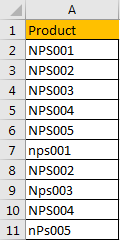## Part 1: Count Duplicate Values Once with Case Sensitive

Step 1: Select a blank cell just next to the list, for example B2, enter the formula =SUM(IFERROR(1/IF(A2:A11<>””, FREQUENCY(IF(EXACT(A2:A11, TRANSPOSE(A2:A11)), MATCH(ROW(A2:A11), ROW(A2:A11)), “”), MATCH(ROW(A2:A11), ROW(A2:A11))), 0), 0)).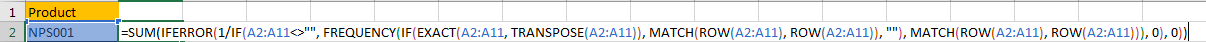Step 2: Press Shift+Ctrl+Enter on keyboard simultaneously to get result.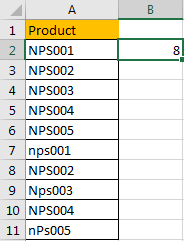As we count number with case sensitive in this sample, so product ‘NPS001’ and ‘nps001’ are processed as two different products.

## Part 2: Count Duplicate Values Once with Case Insensitive

This time we count number with case insensitive.

Step 1: In B2 enter the formula =SUMPRODUCT((A2:A11<>””)/COUNTIF(A2:A11,A2:A11&””)).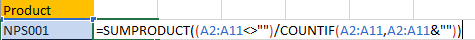Step 2: Press Enter directly to get result. Verify that this time the count number is 5, so this time products ‘NPS001’ and ‘nps001’ are considered as duplicate values.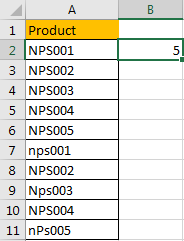### Related Functions

• Excel SUMPRODUCT function
The Excel SUMPRODUCT function multiplies corresponding components in the given one or more arrays or ranges, and returns the sum of those products.The syntax of the SUMPRODUCT function is as below:= SUMPRODUCT (array1,[array2],…)…
• Excel COUNTIF function
The Excel COUNTIF function will count the number of cells in a range that meet a given criteria. This function can be used to count the different kinds of cells with number, date, text values, blank, non-blanks, or containing specific characters.etc.= COUNTIF (range, criteria)…
• Excel SUM function
The Excel SUM function will adds all numbers in a range of cells and returns the sum of these values. You can add individual values, cell references or ranges in excel.The syntax of the SUM function is as below:= SUM(number1,[number2],…)…
• Excel IFERROR function
The Excel IFERROR function returns an alternate value you specify if a formula results in an error, or returns the result of the formula.The syntax of the IFERROR function is as below:= IFERROR (value, value_if_error)….
• Excel IF function
The Excel IF function perform a logical test to return one value if the condition is TRUE and return another value if the condition is FALSE. The IF function is a build-in function in Microsoft Excel and it is categorized as a Logical Function.The syntax of the IF function is as below:= IF (condition, [true_value], [false_value])….
• Excel EXACT function
The Excel EXACT function compares if two text strings are the same and returns TRUE if they are the same, Or, it will return FALSE.The syntax of the EXACT function is as below:= EXACT (text1,text2)…
• Excel MATCH  function
The Excel MATCH function search a value in an array and returns the position of that item.The MATCH function is a build-in function in Microsoft Excel and it is categorized as a Lookup and Reference Function.The syntax of the MATCH function is as below:= MATCH  (lookup_value, lookup_array, [match_type])….
• Excel ROW function
The Excel ROW function returns the row number of a cell reference.The ROW function is a build-in function in Microsoft Excel and it is categorized as a Lookup and Reference Function.The syntax of the ROW function is as below:= ROW ([reference])….